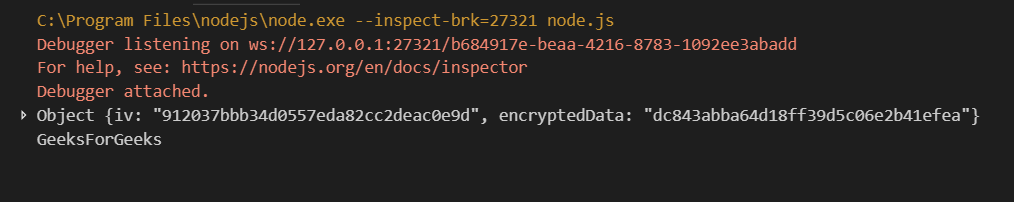# Encrypting Data in NodeJS

Encryption and Decryption in Node can be done by installing and implementing the ‘crypto’ library. If you have installed Node.js by manual build, then there is a chance that the crypto library is not shipped with it. You can run
the following command to install the crypto dependency.

```npm install crypto --save
```

But you don’t need to do that if you have installed it using pre-built packages.

Example for implementing crypto in Node.

 `// Nodejs encryption with CTR ` `const crypto = require(``'crypto'``); ` `const algorithm = ``'aes-256-cbc'``; ` `const key = crypto.randomBytes(32); ` `const iv = crypto.randomBytes(16); ` ` `  `function` `encrypt(text) { ` `let cipher = crypto.createCipheriv(``'aes-256-cbc'``,Buffer.from(key), iv); ` `let encrypted = cipher.update(text); ` `encrypted = Buffer.concat([encrypted, cipher.final()]); ` `return` `{ iv: iv.toString(``'hex'``), encryptedData: encrypted.toString(``'hex'``) }; ` `} ` ` `  `function` `decrypt(text) { ` `let iv = buffer.from(text.iv, ``'hex'``); ` `let encryptedText = Buffer.from(text.encryptedData, ``'hex'``); ` `let decipher = crypto.createDecipheriv(``'aes-256-cbc'``, Buffer.from(key), iv); ` `let decrypted = decipher.upate(encryptedText); ` `decypted = Buffer.concat([decrypted, decipher.final()]); ` `return` `decrypted.toString(); ` `} ` ` `  `var` `gfg = encrypt(``"GeeksForGeeks"``); ` `console.log(gfg); ` `console.log(decrypt(gfg)); `

OutputMy Personal Notes arrow_drop_upCheck out this Author's contributed articles.

If you like GeeksforGeeks and would like to contribute, you can also write an article using contribute.geeksforgeeks.org or mail your article to contribute@geeksforgeeks.org. See your article appearing on the GeeksforGeeks main page and help other Geeks.

Please Improve this article if you find anything incorrect by clicking on the "Improve Article" button below.

Article Tags :

Be the First to upvote.

Please write to us at contribute@geeksforgeeks.org to report any issue with the above content.